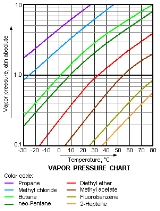xPartial pressureOverview

In a mixture of ideal gas
Ideal gas
An ideal gas is a theoretical gas composed of a set of randomly-moving, non-interacting point particles. The ideal gas concept is useful because it obeys the ideal gas law, a simplified equation of state, and is amenable to analysis under statistical mechanics.At normal conditions such as...

es, each gas has a partial pressure which is the pressure which the gas would have if it alone occupied the volume. The total pressure
Pressure
Pressure is the force per unit area applied in a direction perpendicular to the surface of an object. Gauge pressure is the pressure relative to the local atmospheric or ambient pressure.- Definition :...

of a gas mixture is the sum of the partial pressures of each individual gas in the mixture.

In chemistry
Chemistry
Chemistry is the science of matter, especially its chemical reactions, but also its composition, structure and properties. Chemistry is concerned with atoms and their interactions with other atoms, and particularly with the properties of chemical bonds....

, the partial pressure of a gas
Gas
Gas is one of the three classical states of matter . Near absolute zero, a substance exists as a solid. As heat is added to this substance it melts into a liquid at its melting point , boils into a gas at its boiling point, and if heated high enough would enter a plasma state in which the electrons...

in a mixture of gases is defined as above. The partial pressure of a gas dissolved in a liquid is the partial pressure of that gas which would be generated in a gas phase in equilibrium with the liquid at the same temperature.